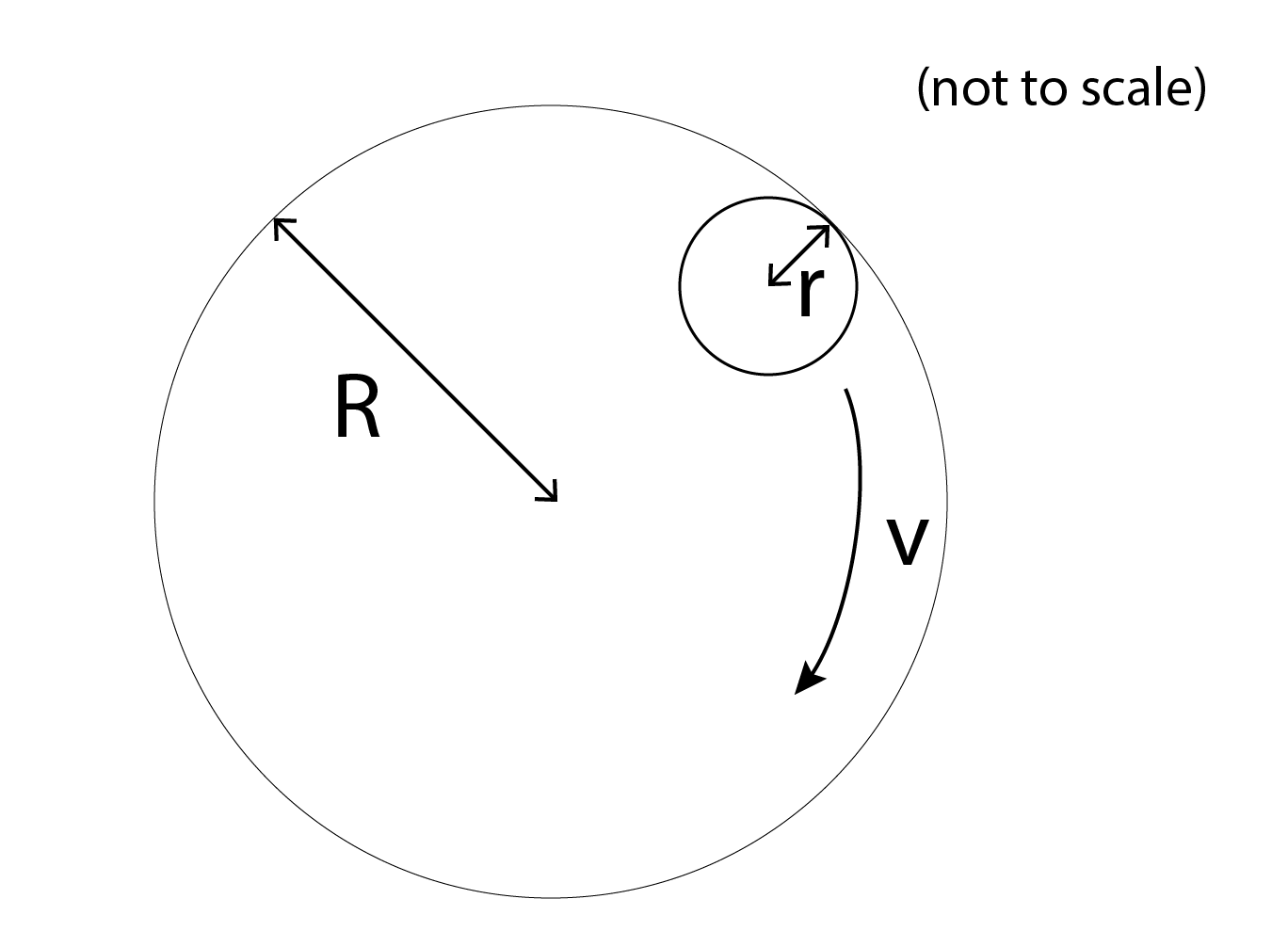# Circular Motion #5A sphere of mass $m$, radius $r$ moves in a circle with constant speed $v$, always touching the inner surface of a fixed hollow cylinder with radius $R$. The top view of the system is shown. All surfaces are smooth. If the magnitude of the normal reaction (in Newtons) exerted by the cylinder on the sphere is $\frac{ A } { B }$ where $A$ and $B$ are co-prime positive integers, what is $A + B$?

• $m = 0.1 \text{ kg}$
• $v = 1 \text{ m s}^{-1}$
• $r = 0.1 \text{ m}$
• $R = 1 \text{ m}$

This problem is part of the set - Circular Motion Practice

×

Problem Loading...

Note Loading...

Set Loading...